﻿ 暗物质是能量场气场物质，类似电场磁场物质

# 暗物质是能量场气场物质，类似电场磁场物质Dark Matter Is the Energy Field and Qi Field Substance, Similar to the Electric Field and Magnetic Field Substance

Abstract: Space-time ladder theory reveals that the matter (energy) changes can generate Energy-Qi field, and dark matter is present in the vicinity of matter (galaxy). Therefore, the first speculation of dark matter is the Energy-Qi field. Through comparative study, we found that in addition to the Newtonian gravity, the movement of the stars is also affected by the energy field and the Qi field:, where F is the force of Energy-Qi field, m is the mass of the stars, E is the energy field strength, v is the speed of the stars, and Q is the Qi induction. And the moving speed of the stars is:, that is, the spiral galaxy rotation curve increases with the distance increasing, just offsetting the Kepler’s law which the speed of the stars decrease with square root of the dis-tance increasing. If there is any complement to Newton’s gravitation, this is a complement of New-ton’s gravitation: In addition to the mass m that can cause gravitation, two new concepts have emerged here: The energy field and the Qi field can also cause gravitation. These two concepts appeared in the Maxwell’s equations when we compared the energy and the Chinese medicine Qi. In fact, this new Maxwell equations can be called the Energy-Qi field equations. With the Energy-Qi field equations, combined with the Lorentz force derived through the analogy research, we can describe and calculate the dark matter. Surprisingly, we can deduce the third law of Kepler by the equation obtained by analogy research and the Newton’s gravitation equation, and the result is the same. This proves indirectly that the equations derived from the analogy research are correct. At this point, dark matters finally show their true colors: The Energy-Qi field, and the true appearance of dark matter is obtained by its compressed version of the electric field and magnetic field. Because the electric and magnetic fields can be easily detected, but the energy field and the Qi field are too weak and hard to be detected. Therefore, through comparative studies, we finally find the root cause of the Galaxy rotation curve problem: The Energy-Qi field, that is, the dark matter we are looking for. In addition, through the comparison of the Energy-Qi field and the Electromagnetic field, we found out the principle of the future means of transport, laying a theoretical foundation for us to truly enter the universe.

1. 引言

2. 历史回顾

1) 能量场是由能量生成。能量线开始于能量收缩态，终止于能量膨胀态。能量收缩态就是原子核状态，能量膨胀态就是暗能量。

2) 气场线没有初始点，也没有终止点。气场线会形成循环或延伸至无穷远。换句话说，进入任何区域的气场线，也必须从那区域离开。通过任意闭曲面的气通量等于零，气场是一个螺线矢量场。

3) 描述随时间变化的气场生成能量场。

4) 气场可以用两种方法生成：一种是靠能量流产生，另一种是靠随时间变化的能量场产生。

${F}_{1}=q\left({E}_{1}+{v}_{1}×B\right)$

$F=m\left(E+v×Q\right)$ ，(可以称之为洛伦兹力能气方程)。

$R=\frac{mv\mathrm{sin}\theta }{Bq}$ ,

$T=\frac{2\text{π}m}{Bq}$ ,

$h=\frac{2\text{π}mv\mathrm{cos}\theta }{Bq}$ .

$R=\frac{mv\mathrm{sin}\theta }{Qm}=\frac{v\mathrm{sin}\theta }{Q}$ ,

$T=\frac{2\text{π}m}{Qm}=\frac{2\text{π}}{Q}$ ,

$h=\frac{2\text{π}mv\mathrm{cos}\theta }{Qm}=\frac{2\text{π}v\mathrm{cos}\theta }{Q}$ .

$F=\frac{m{v}^{2}}{R}=\frac{GMm}{{R}^{2}}$

$v=\sqrt{\frac{GM}{R}}$

$v=\frac{RQ}{\mathrm{sin}\theta }$

$v=\sqrt{\frac{GM}{R}}=\frac{RQ}{\mathrm{sin}\theta }$

$\frac{GM}{R}=\frac{{R}^{2}{Q}^{2}}{\mathrm{sin}{\theta }^{2}}$

$T=\frac{2\text{π}m}{Qm}=\frac{2\text{π}}{Q}$

$\frac{GM}{R}=\frac{{R}^{2}{Q}^{2}}{\mathrm{sin}{\theta }^{2}}=\frac{4{\text{π}}^{2}{R}^{2}}{\mathrm{sin}{\theta }^{2}{T}^{2}}$

$\frac{{T}^{2}}{{R}^{3}}=\frac{4{\text{π}}^{2}}{GM\mathrm{sin}{\theta }^{2}}$

$T=\frac{2m\text{π}ab}{\mathcal{l}}$

(远拱点B与引力中心之间的距离)的关系分别为  ：

$a=\frac{{r}_{A}+{r}_{B}}{2}$

$b=\sqrt{{r}_{A}{r}_{B}}$ ,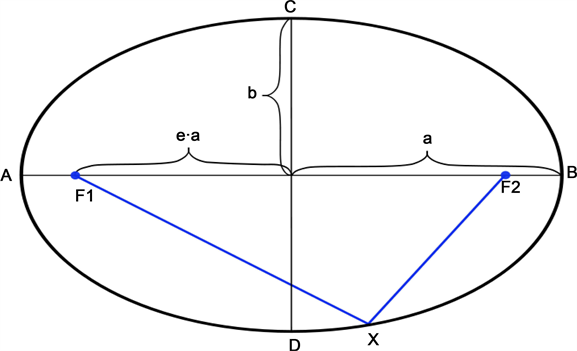Figure 1. Planets orbit about the sun in elliptical orbits (focal point F1)

$E=\frac{1}{2}m{\stackrel{˙}{r}}^{2}+\frac{1}{2}m{r}^{2}{\stackrel{˙}{\theta }}^{2}-G\frac{mM}{r}$ .

$\stackrel{˙}{r}=0$

$E=\frac{1}{2}m{r}^{2}{\stackrel{˙}{\theta }}^{2}-G\frac{mM}{r}=\frac{{\mathcal{l}}^{2}}{2m{r}^{2}}-G\frac{mM}{r}$

${r}^{2}+\frac{GmM}{E}r-\frac{{\mathcal{l}}^{2}}{2mE}=0$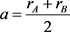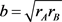,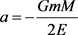,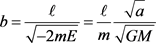.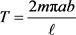,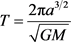,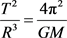,

3. 对牛顿引力的补充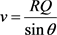，v是星体的运动速度，R是星体距离核心的距离，Q是气场，假如气场是稳定的，夹角也是稳定的，那么星体的运动速度与距离成正比，也就是说，这个效应，正好抵消了开普勒定律效应，正好解决了星系自转曲线问题  。

4. 回旋加速器与未来宇宙交通运输工具

1930年，Earnest O. Lawrence提出了回旋加速器的理论  。回旋加速器(Cyclotron)：它是利用磁场和电场共同使带电粒子作回旋运动，在运动中经高频电场反复加速的装置。

5. 总结

 Dark Matter. CERN Physics. 20 January 2012.

 常炳功. 能量与中医气的关系类似电与磁的关系(现代物理201803).

 McHutchon, A. (2013) Electromagnetism Laws and Equations. Michaelmas 2013.

 开普勒定律. 维基百科, 自由的百科全书.

 Purcell, E.M. and Morin, D.J. (2013) Electricity and Magnetism. 3rd Edition, Cambridge University Press, New York. 820 p.

 Jiles, D.C. (1998) Introduction to Magnetism and Magnetic Materials. 2nd Edition, CRC.

 Corbelli, E. and Salucci, P. (2000) The Extended Rotation Curve and the Dark Matter Halo of M33. Monthly Notices of the Royal Astronomical Society, 311, 441-447.

 Lawrence, E.O. Method and Apparatus for the Acceleration of Ions, Filed: January 26, 1932, Granted: February 20, 1934.

Top